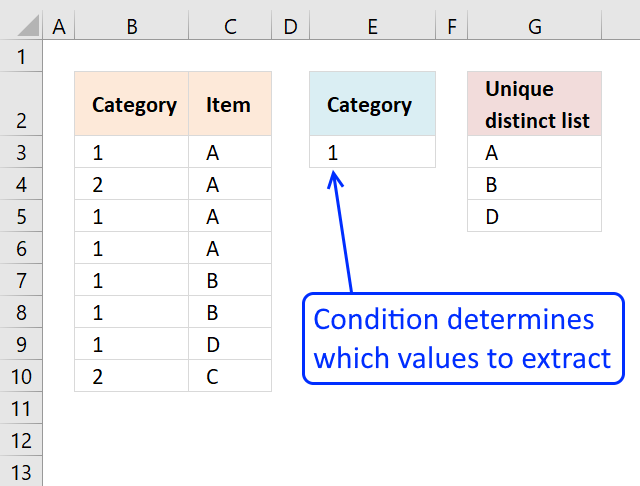Author: Oscar Cronquist Article last updated on October 12, 2018

How to return multiple values using vlookup in excel and removing duplicates?
I have tried the formula to return multiple values using the index example and worked fine with none duplicate item but how can i list them without the duplicate?

The following array formula is easier to understand than a VLOOKUP formula.Update, 2017-08-16! New smaller regular formula.

Formula in cell G3:

=LOOKUP(2, 1/((COUNTIF(\$G\$2:G2, \$C\$3:\$C\$10)=0)*(\$E\$3=\$B\$3:\$B\$10)), \$C\$3:\$C\$10)

Array formula in cell G3:

=INDEX(\$C\$3:\$C\$10,MATCH(0,COUNTIF(\$G\$2:G2,\$C\$3:\$C\$10)+(\$B\$3:\$B\$10<>\$E\$3),0))

The formulas above do not sort the unique distinct list.

#### Watch I video where I explain the formula above

Recommended articles

5 easy ways to extract Unique Distinct Values

First, let me explain the difference between unique values and unique distinct values, it is important you know the difference […]

5 easy ways to VLOOKUP and return multiple values

This post explains how to lookup a value and return multiple values. No array formula required.

Use VLOOKUP and return multiple values sorted from A to Z

This article demonstrates how to extract multiple values based on a search value and display them sorted from A to […]

#### How to enter an array formula

1. Double press with left mouse button on cell G3
2. Copy (Ctrl + c) and paste (Ctrl + v) array formula to cell
3. Press and hold Ctrl + Shift simulatenously
4. Press Enter once
5. Release all keys

A beginners guide to Excel array formulas

Array formulas allows you to do advanced calculations not possible with regular formulas.

#### Copy array formula

1. Select cell G3
2. Copy cell ( Ctrl + c)
3. Select cell range G4:10
4. Paste (Ctrl - v)

#### Explaining array formula in cell E8

Step 1 - Compare cell value in E3 with column Category and return a boolean array

\$B\$3:\$B\$10<>\$E\$3

becomes

{1;2;1;1;1;1;1;2}<>1

and returns {FALSE; TRUE; FALSE; FALSE; FALSE; FALSE; FALSE; TRUE}

Step 2 - Check earlier values above current cell

COUNTIF(\$G\$2:G2,\$C\$3:\$C\$10)

becomes

COUNTIF("Unique distinct list",{"A";"A";"A";"A";"B";"B";"D";"C"})

and returns {0;0;0;0;0;0;0;0}

How to use the COUNTIF function

Counts the number of cells that meet a specific condition.

COUNTIF(\$G\$2:G2,\$C\$3:\$C\$10)+(\$B\$3:\$B\$10<>\$E\$3)

becomes

{FALSE; TRUE; FALSE; FALSE; FALSE; FALSE; FALSE; TRUE} + {0;0;0;0;0;0;0;0}

and returns {0;1;0;0;0;0;0;1}

Step 4 - Match first zero value in array and return relative position

MATCH(0,COUNTIF(\$G\$2:G2,\$C\$3:\$C\$10)+(\$B\$3:\$B\$10<>\$E\$3),0)

becomes

MATCH(0,{0;1;0;0;0;0;0;1},0)

and returns 1.

How to use the MATCH function

Identify the position of a value in an array.

Step 5 - Return a value or reference of the cell at the intersection of a particular row and column

INDEX(\$C\$3:\$C\$10,MATCH(0,COUNTIF(\$G\$2:G2,\$C\$3:\$C\$10)+(\$B\$3:\$B\$10<>\$E\$3),0))

becomes

INDEX(\$C\$3:\$C\$10,1)

becomes

INDEX({"A";"A";"A";"A";"B";"B";"D";"C"},1)

and returns "A" in cell G3.

How to use the INDEX function

Gets a value in a specific cell range based on a row and column number.

#### Get excel file

Vlookup - Return multiple unique distinct valuesv2.xlsx
(Excel 97-2003 Workbook *.xls)